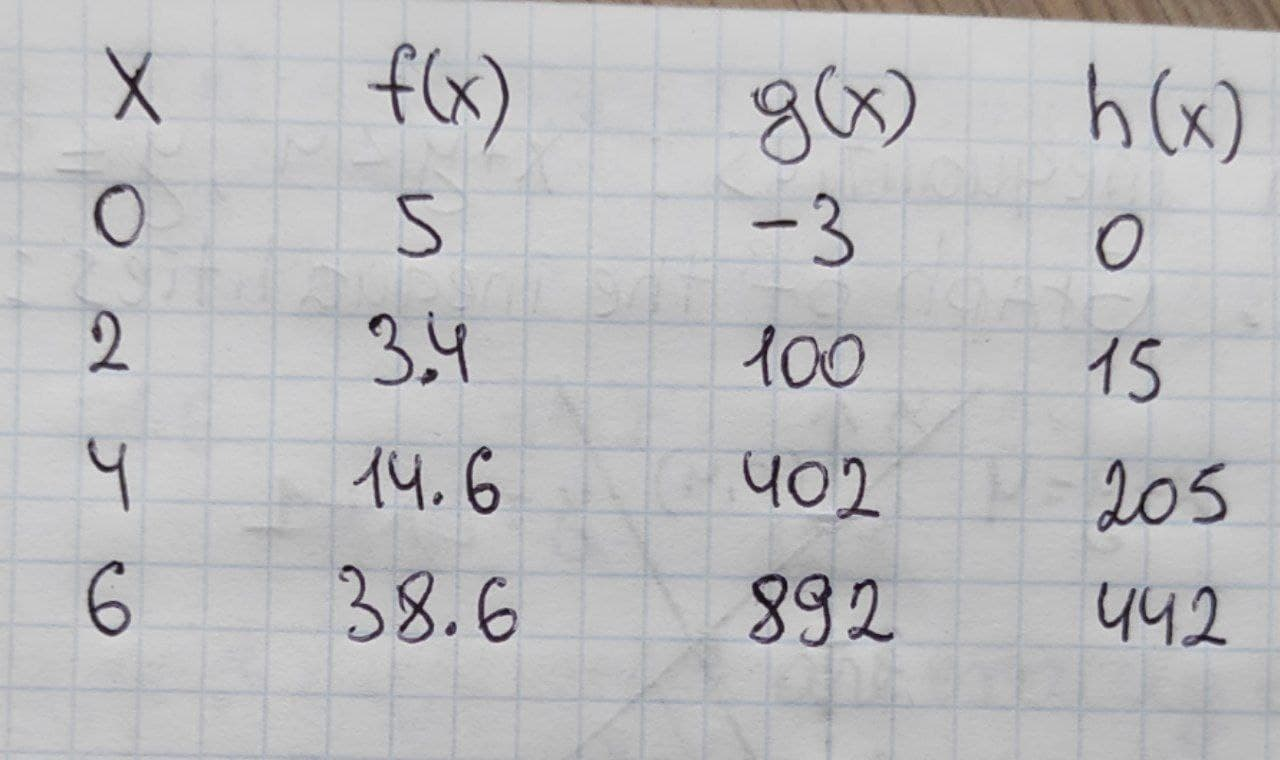# Get help with quadratics practice problems### Find the complex zeros of the following polynomial function. Write f in factored form. f(x)=x3-8

ANSWERED### Give all possible answers $$\displaystyle{m}\angle{C}={36}^{{\circ}},\ {A}{B}={31},\ {B}{C}={24},$$ find $$\displaystyle{m}\angle{A}$$

ANSWERED### Prove that: 1. $$\displaystyle{2}{a}{b}\le{a}^{{2}}+{b}^{{2}}$$ 2. $$\displaystyle{a}{b}+{a}{c}+{b}{c}\le{a}^{{2}}+{b}^{{2}}+{c}^{{2}}$$ If a, b and c are all integers.

ANSWERED### Three fire observation towers are located at points A (-6,-12), B (11,15), and C (-3,15), on a map where all the units are in kilometers. A fire is located at distances of 17 km, 15km, and 13km, respectively, from the observation towers. Graph three center circles whose centers are located at the observation towers and whose radii are the given distances to the fire. Then estimate the location of the fire.

ANSWERED### Simplify the following expression: -6i(3-2i)

ANSWERED### Find the value of the following expression in terms of a+bi: $$\displaystyle\frac{\sqrt{{-{6}}}}{{\sqrt{{-{3}}}\sqrt{{-{4}}}}}$$

ANSWERED### Use the quadratic formula $$\displaystyle{y}^{{{2}}}-{7}{y}-{5}={0}$$

ANSWERED### Find two positive numbers whose sum is 676 and the sum of whose squares is a minimum.

ANSWERED### Prove that $$\displaystyle-{3}^{{4}}\ne{\left(-{3}\right)}^{{4}}$$

ANSWERED### P varies directly as x and inversely as the square of y. $$\displaystyle{d}{i}{s}{p}{l}{a}{y}{s}{t}{y}\le{\left\lbrace{\left({\left\lbrace{P}\right\rbrace}={\frac{{{28}}}{{{\left\lbrace{3}\right\rbrace}}}}{\left\lbrace{w}\right\rbrace}{\left\lbrace{h}\right\rbrace}{\left\lbrace{e}\right\rbrace}{\left\lbrace{n}\right\rbrace}{\left\lbrace{x}\right\rbrace}={\left\lbrace{42}\right\rbrace}{\left\lbrace\quad\text{and}\quad\right\rbrace}{\left\lbrace{y}\right\rbrace}={\left\lbrace{9}\right\rbrace}\right)}\right\rbrace}$$

ANSWERED### Find the value of the following expression: $$\displaystyle{3}\sqrt{{{64}{\left({x}+{6}\right)}}}$$

ANSWERED### Solve the following quadratic equation $$\displaystyle{3}{x}^{{2}}+{5}{x}+{2}={0}$$

ANSWERED### Deetermine, without graphing, ehether the given quaratic function has a maximum value or a minimum value and then find the valur. $$f(x) = 2x^2-12x$$

ANSWERED### Simplify: $$\displaystyle{2}\sqrt{{{8}}}\sqrt{{{18}}}$$

ANSWERED### Find the roots of the function $$\displaystyle{f{{\left({x}\right)}}}={\left({2}{x}−{1}\right)}\cdot{\left({x}^{{2}}+{2}{x}−{3}\right)}$$, with $$\displaystyle{x}\in{R}$$

ANSWERED### Solve the quadratic equation for all values of x in simpls form $$\displaystyle{4}{\left({x}+{8}\right)}^{{2}}={4}$$

ANSWERED### Express $$\displaystyle{x}^{{{2}}}+{8}{x}$$  in the form  $$\displaystyle{\left({x}+{a}\right)}{2}+{b}$$

ANSWERED### Find the vertex of the qudratic function $$\displaystyle{f{{\left({x}\right)}}}={x}^{{2}}-{6}{x}+{42}$$, then express the qudratic function in standart form $$\displaystyle{f{{\left({x}\right)}}}={a}{\left({x}-{h}\right)}^{{2}}+{k}$$ and state whether the vertex is a minimum or maximum. Enter exat answers only, no approximations.

ANSWERED### The table to the right has the​ inputs, x, and the outputs for three​ functions, f,​ g, and h. Use second differences to determine which function is exactly​ quadratic, which is approximately​ quadratic, and which is not quadratic.The functon f(x) is _______ quadratic, g(x) is _____ quadratic, h(x) is _______ quadratic.
ANSWERED